Next: Calorimetry Up: Detector Previous: Vertexing

## Tracking

Once the required momentum resolution is determined, the scale of the tracking detector is calculated by the following well-known equation: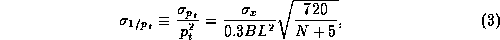where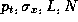and B are transverse momentum (GeV), spatial resolution(m), length(m) of measuring a trajectory, number of sampling points and solenoid field(Tesla).

As mentioned before, the JLC group set the momentum resolution based on "the recoil mass resolution of light Higgs boson = beam energy spread of 200MeV", that is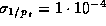. L was calculated to be 2m with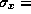100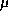m, N=100(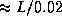) of a conventional drift chamber comprizing small jet-cells and B=2Tesla. The outer radius turned out to be 2.3m with an inner radius of 0.3m (Fig.13). The wires are thus 4.6m long so as to cover an angular region of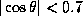for full sampling. Because of such long wires, there are R&D issues concerning the stability of the wire tension, the gravitational and electrostatic sags and so on.

N.Khalatyan presented the results concerning the JLC-CDC test chamber, which is 30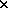30cmand 4.6m long and comprises 5 small cells.  The tension of all field wires, which are 120m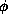gold-plated aluminum, has been decreased by 10% over a period of one year. The reason was considered to be the material, itself, because of the uniform lengthening. Thus, a good material must be found. The gravitational sags were measured to be 355m and 600m for sense wires of 30mgold-plated tungsten and the field wires, respectively. These values are in good agreement with the calculations. The electrostatic sag of the sense wires was also measured to be 110m, which fairly agrees with the calculated value of 85m. These sags can thus be corrected to achieve the designed resolution.

In order to separate the background trajectories coming from neighboring bunch crossings, the time resolution is crucial. If a charged particle hits all 100 wires, the time resolution for the trajectory can be obtained to be 10nsec/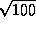=1nsec because of the drift velocity of 10m/nsec. Therefore, the originated bunch can be identified for a bunch spacing of 1.45.6 nsec for JLC-I.Next: Calorimetry Up: Detector Previous: Vertexing

Toshiaki Tauchi
Fri, Dec 20, 1996 02:24:05 PM